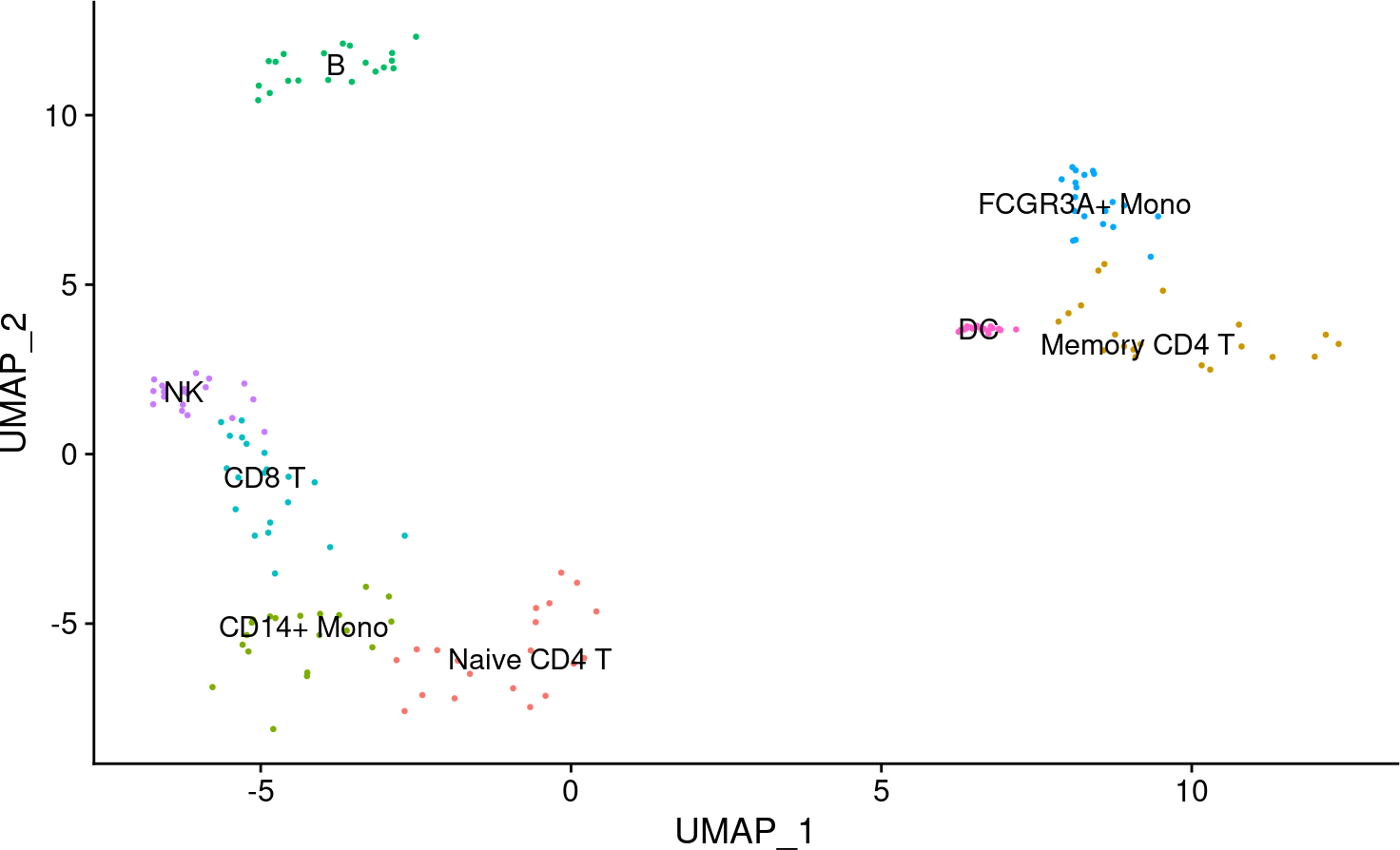scRNA-seq yield many molecular readouts that are hard to interpret by themselves. One way of summarizing this information is by inferring pathway activities from prior knowledge.

In this notebook we showcase how to use `decoupleR` for pathway activity inference with a down-sampled PBMCs 10X data-set. The data consists of 160 PBMCs from a Healthy Donor. The original data is freely available from 10x Genomics here from this webpage.

First, we need to load the relevant packages, `Seurat` to handle scRNA-seq data and `decoupleR` to use statistical methods.

``````## We load the required packages
library(Seurat)
library(decoupleR)

# Only needed for data handling and plotting
library(dplyr)
library(tibble)
library(tidyr)
library(patchwork)
library(ggplot2)
library(pheatmap)``````

Here we used a down-sampled version of the data used in the `Seurat` vignette. We can open the data like this:

``````inputs_dir <- system.file("extdata", package = "decoupleR")

We can observe that we have different cell types:

``DimPlot(data, reduction = "umap", label = TRUE, pt.size = 0.5) + NoLegend()``# 3 PROGENy model

PROGENy is a comprehensive resource containing a curated collection of pathways and their target genes, with weights for each interaction. For this example we will use the human weights (mouse is also available) and we will use the top 100 responsive genes ranked by p-value. We can use `decoupleR` to retrieve it from `OmniPath`:

``````net <- get_progeny(organism = 'human', top = 100)
net
#> # A tibble: 1,400 × 4
#>    source   target  weight  p_value
#>    <chr>    <chr>    <dbl>    <dbl>
#>  1 Androgen TMPRSS2  11.5  2.38e-47
#>  2 Androgen NKX3-1   10.6  2.21e-44
#>  3 Androgen MBOAT2   10.5  4.63e-44
#>  4 Androgen KLK2     10.2  1.94e-40
#>  5 Androgen SARG     11.4  2.79e-40
#>  6 Androgen SLC38A4   7.36 1.25e-39
#>  7 Androgen MTMR9     6.13 2.53e-38
#>  8 Androgen ZBTB16   10.6  1.57e-36
#>  9 Androgen KCNN2     9.47 7.71e-36
#> 10 Androgen OPRK1    -5.63 1.11e-35
#> # ℹ 1,390 more rows``````

# 4 Activity inference with Weighted Mean

To infer activities we will run the Weighted Mean method (`wmean`). It infers regulator activities by first multiplying each target feature by its associated weight which then are summed to an enrichment score `wmean`. Furthermore, permutations of random target features can be performed to obtain a null distribution that can be used to compute a z-score `norm_wmean`, or a corrected estimate `corr_wmean` by multiplying `wmean` by the minus log10 of the obtained empirical p-value.

In this example we use `wmean` but we could have used any other. To see what methods are available use `show_methods()`.

To run `decoupleR` methods, we need an input matrix (`mat`), an input prior knowledge network/resource (`net`), and the name of the columns of net that we want to use.

``````# Extract the normalized log-transformed counts
mat <- as.matrix(data@assays\$RNA@data)

# Run wmean
acts <- run_wmean(mat=mat, net=net, .source='source', .target='target',
.mor='weight', times = 100, minsize = 5)
acts
#> # A tibble: 6,720 × 5
#>    statistic  source   condition           score p_value
#>    <chr>      <chr>    <chr>               <dbl>   <dbl>
#>  1 corr_wmean Androgen AAACATACAACCAC-1  0.00321  0.94
#>  2 corr_wmean Androgen AAACGCTGTTTCTG-1 -0.0147   0.34
#>  3 corr_wmean Androgen AACCTTTGGACGGA-1  0        0.0800
#>  4 corr_wmean Androgen AACGCCCTCGTACA-1  0        0.0200
#>  5 corr_wmean Androgen AACGTCGAGTATCG-1  0.0242   0.140
#>  6 corr_wmean Androgen AACTCACTCAAGCT-1  0.00672  0.0800
#>  7 corr_wmean Androgen AAGATGGAAAACAG-1  0.0250   0.62
#>  8 corr_wmean Androgen AAGATTACCGCCTT-1  0.00849  0.86
#>  9 corr_wmean Androgen AAGCCATGAACTGC-1  0.0314   0.58
#> 10 corr_wmean Androgen AAGGTCTGCAGATC-1  0        0.5
#> # ℹ 6,710 more rows``````

# 5 Visualization

From the obtained results, we will select the `norm_wmean` activities and store them in our object as a new assay called `pathwayswmean`:

``````# Extract norm_wmean and store it in pathwayswmean in data
data[['pathwayswmean']] <- acts %>%
filter(statistic == 'norm_wmean') %>%
pivot_wider(id_cols = 'source', names_from = 'condition',
values_from = 'score') %>%
column_to_rownames('source') %>%
Seurat::CreateAssayObject(.)

# Change assay
DefaultAssay(object = data) <- "pathwayswmean"

# Scale the data
data <- ScaleData(data)
data@assays\$pathwayswmean@data <- data@assays\$pathwayswmean@scale.data``````

This new assay can be used to plot activities. Here we visualize the Trail pathway, associated with apoptosis, which seems that in B and NK cells is more active.

``````p1 <- DimPlot(data, reduction = "umap", label = TRUE, pt.size = 0.5) +
NoLegend() + ggtitle('Cell types')
p2 <- (FeaturePlot(data, features = c("Trail")) &
scale_colour_gradient2(low = 'blue', mid = 'white', high = 'red')) +
ggtitle('Trail activity')
p1 | p2``````